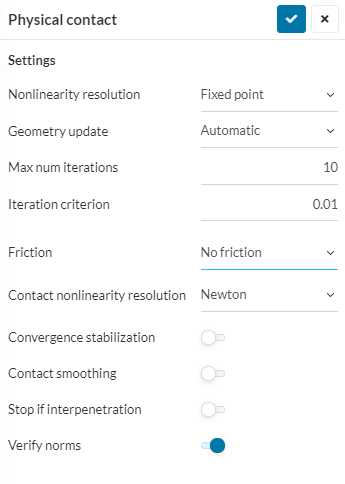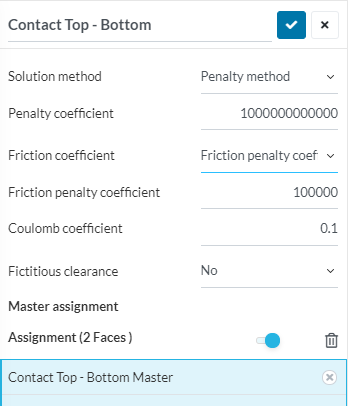# Setting a coefficient of friction

When doing a non-linear thermomechanical study, we have the option of choosing a friction interface between bodies but I can not see where to define the coefficient of friction? I do understand that since the thermomechanical solver first solves for temperature and then for forces, the friction functionality is limited, but still, I don’t understand how it can calculate a frictional force without a coefficient of friction

Hi Roy and sorry for the late response!

Some pictures to demonstrate how you would set up/ find the Coulomb Coefficient:

\underline{\text{1. Set up a Physical Contact}}\underline{\text{2. Set Coulomb Coefficient}}Is that what you were looking for? Let me know if you need anything else, happy to help you out!

Jousef

1 Like

Hi @jousefm

Thanks for the reply. I didn’t get as far as the 2nd screen so did not see that. What is the penalty coefficient and friction penalty coefficient?

Hi Roy!

As for the difference the penalty method produces a small interpenetration whereas the Augmented Lagrange method is quite exact (almost). The penalty coefficient is something between 1-100x the Young’s Modulus of your material (anything too big will lead to an ill-conditioned matrix). In the same way the friction penalty coefficient is assigned to the tangential direction. The penalty parameter is a scaling factor to calculate the normal pressure, but only when there is contact or interpenetration.

Cheers,

Jousef

1 Like